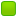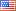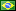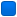###Author Topic: How are you multiplying by 3  (Read 822 times)

####gygzkunw

• Jr. Member
•• Posts: 8##### How are you multiplying by 3
« on: February 22, 2021, 12:21:15 AM »
Code: [Select]
`SECTION .bss BUFFLEN equ 16 ; ----> Bufflen = 16 Buff: resb BUFFLEN ; ----> Buff will read 16 bytes from the buffer SECTION .data ; Section containing initialised data HexStr: db " 00 00 00 00 00 00 00 00 00 00 00 00 00 00 00 00",10 HEXLEN equ \$-HexStr ; ---->length of Hexstr Digits: db "0123456789ABCDEF" SECTION .text ; Section containing codeglobal _start ; Linker needs this to find the entry point! _start: nop ; This no-op keeps gdb happy...; Read a buffer full of text from stdin:Read: mov eax,3 ; ----> syscall read mov ebx,0 ; ----> read from standard input (keyboard) mov ecx,Buff ; ----> where the string read is stored mov edx,BUFFLEN ; ----> how many bytes is supposed to read int 80h ; ----> sys call mov ebp,eax ; ----> copy the number that where actually read into ebp cmp eax,0 ; ----> sub 0 from eax and set the appropriate flag? (if user enters 0 shouldn't the program end?) je Done ; ----> if the zero flag is set move tone the label done; Set up the registers for the process buffer step: mov esi,Buff ; ----> copy the content of Buff into esi mov edi,HexStr ; ----> copy hexstr into edi xor ecx,ecx ; ----> Clears ecx; Go through the buffer and convert binary values to hex digits:Scan:s xor eax,eax ; ----> clears eax; Here we calculate the offset into the line string, which is ecx X 3 mov edx,ecx ; ----> copy ecx which is 0 into edx lea edx,[edx*2+edx]     ; ----> This is where i am stuck. How are you able to multiply by 3 over here? LEA = [BASE +( INDEX * SCALE) + DISP)]       if edx is 0, how are we able to multiply by 3`
I am not understand how the author is using the lea instruction
The code is from Assembly Language Step-By-Step by Jeff Duntemann

Thank you
« Last Edit: February 22, 2021, 01:56:10 AM by gygzkunw »

####Frank Kotler

• NASM Developer
• Hero Member
•• Posts: 2482
• Country:##### Re: How are you multiplying by 3
« Reply #1 on: February 22, 2021, 03:07:34 AM »
Well... it's a "trick" using lea (load effective address) to "load"  edx with edx plus edx times two... As you observe, zero times three is zero. You don't show it, but we then increment edx.. and ,,,

I've never read "Step by Step"... I ASSume Jeff explains this...?

Best,
Frank

####gygzkunw

• Jr. Member
•• Posts: 8##### Re: How are you multiplying by 3
« Reply #2 on: February 22, 2021, 04:27:45 AM »
Well... it's a "trick" using lea (load effective address) to "load"  edx with edx plus edx times two... As you observe, zero times three is zero. You don't show it, but we then increment edx.. and ,,,

I've never read "Step by Step"... I ASSume Jeff explains this...?

Best,
Frank
I get its a trick but i don't see where the 3 is coming from...

"Not only is this virtually always faster than shifts combined with adds, it’s also clearer from your source code what sort of calculation is actually being done. The fact that what ends up in EDX may not in fact be the legal address of anything is unimportant.LEA does not try to reference the address it calculates.It does the math on the stuff inside the brackets and drops it into the destination operand. Job over. Memory is not touched, and the flags are not affected.Of  course,  you’re  limited  to  what  calculations  can  be  done  on  effective addresses; but right off the top, you can multiply any GP register by 2, 3, 4, 5, 8,and 9, while tossing in a constant too! It’s not arbitrary math, but multiplying by  2,  3,  4,  5,  8,  and  9  comes  up  regularly  in  assembly  work,  and  you  can combine LEA with shifts and adds to do more complex math and ‘‘fill in the holes.’’ You can also use multiple LEA instructions in a row."

Is there a book you like to recommend? You dont seem to like the book...
« Last Edit: February 22, 2021, 04:31:37 AM by gygzkunw »

####Frank Kotler

• NASM Developer
• Hero Member
•• Posts: 2482
• Country:##### Re: How are you multiplying by 3
« Reply #3 on: February 22, 2021, 05:21:03 AM »
edx plus two times edx  equals three times edx. I don't think I understand the question.

I have not read Jeff Duntemann's book. I did not mean to give the impression I didn't like it! It has an excellent reputation. I don't have another suggestion. Dr. Paul Carter's Tutorial, perhaps?

Best,
Frank

####gygzkunw

• Jr. Member
•• Posts: 8##### Re: How are you multiplying by 3
« Reply #4 on: February 22, 2021, 02:11:02 PM »
edx+2 * edx = 3edx-> This is my question, how is this possible?

But earlier mov edx,ecx -> 0 was moved into edx

edx(0)+ 2*edx(0) =should equal to 0, right?
Do you understand what I am saying here?

####fredericopissarra

• Full Member
•• Posts: 142
• Country:##### Re: How are you multiplying by 3
« Reply #5 on: February 22, 2021, 03:40:15 PM »
edx+2 * edx = 3edx-> This is my question, how is this possible?

EDX + 2*EDX = EDX * (1 + 2) = EDX * 3

Elementary arithmetic.

Quote
But earlier mov edx,ecx -> 0 was moved into edx

edx(0)+ 2*edx(0) =should equal to 0, right?
Do you understand what I am saying here?
Yep... this code is obviously WRONG. Or, at least, is incomplete (and ECX is updated in a loop).
« Last Edit: February 22, 2021, 03:43:50 PM by fredericopissarra »

####gygzkunw

• Jr. Member
•• Posts: 8##### Re: How are you multiplying by 3
« Reply #6 on: February 22, 2021, 04:34:33 PM »
edx+2 * edx = 3edx-> This is my question, how is this possible?

EDX + 2*EDX = EDX * (1 + 2) = EDX * 3

Elementary arithmetic.

Quote
But earlier mov edx,ecx -> 0 was moved into edx

edx(0)+ 2*edx(0) =should equal to 0, right?
Do you understand what I am saying here?
Yep... this code is obviously WRONG. Or, at least, is incomplete (and ECX is updated in a loop).
I didn't say it wasn't elementary. To get 3 you have to add 1. The question is where does the 1 come from? Up till this point in the author does not explain it.

####debs3759

• Global Moderator
• Full Member
•• Posts: 122
• Country:##### Re: How are you multiplying by 3
« Reply #7 on: February 22, 2021, 07:04:20 PM »
I can't figure out what you are not seeing? (EDX * 2) + EDX is obviously 3 * EDX, where are you seeing the "1" that you don't understand?

0 can be multiplied by any number...
My graphics card database: www.gpuzoo.com

####mik3ca

• Jr. Member
•• Posts: 30##### Re: How are you multiplying by 3
« Reply #8 on: February 27, 2021, 01:56:47 AM »
Code: [Select]
`...; Here we calculate the offset into the line string, which is ecx X 3 mov edx,ecx ; ----> copy ecx which is 0 into edx lea edx,[edx*2+edx]     ; ----> This is where i am stuck. How are you able to multiply by 3 over here? LEA = [BASE +( INDEX * SCALE) + DISP)]       if edx is 0, how are we able to multiply by 3`

Simplest way to multiply by 3 is this

Quote
mov EAX,3
mul ECX

It will give you the result as EDX:EAX but if the value is less than roughly 4 million (whatever 2 to the exponent of 32 is) then you can ignore EAX.

But what I would recommend is to use any register ending in BX or BP because then you can directly address the offset of a string.

The way I directly address a string using a multiply by 3 idea (assuming the addressing model is a modern model) is as follows and here we assume the string is small (what normal string would be 4 million bytes anyway?):

Code: [Select]
`baseoffset equ 666h ;location of the entire string (I just picked 666h as an example)mov EAX,3           ;multiplier valuemul EBX               ;EAX times EBX = EDX:EAXmov EBX,EAX     ;set EBX as result`
Now if you use the above code, and add this code, you can set a byte to the string:
Code: [Select]
`mov DL,77h ;77h is a random byte value I chosemov [baseoffset+EBX],DL ;inject it directly into the string location`
Or replace the above two lines with the line below if you want to read a byte:
Code: [Select]
`mov DL,[baseoffset+EBX]`

####debs3759

• Global Moderator
• Full Member
•• Posts: 122
• Country:##### Re: How are you multiplying by 3
« Reply #9 on: February 27, 2021, 03:26:01 AM »
Code: [Select]
`...; Here we calculate the offset into the line string, which is ecx X 3 mov edx,ecx ; ----> copy ecx which is 0 into edx lea edx,[edx*2+edx]     ; ----> This is where i am stuck. How are you able to multiply by 3 over here? LEA = [BASE +( INDEX * SCALE) + DISP)]       if edx is 0, how are we able to multiply by 3`

Simplest way to multiply by 3 is this

Quote
mov EAX,3
mul ECX

It will give you the result as EDX:EAX but if the value is less than roughly 4 million (whatever 2 to the exponent of 32 is) then you can ignore EAX.

Doing that, you are destroying the contents of other registers than the one you want to multiply, and you are creating longer and slower code.

Quote
But what I would recommend is to use any register ending in BX or BP because then you can directly address the offset of a string.

In 16-bit code, you can use BX or BP as the base, and SI or DI as the index. In 32-bit code you can use any 32-bit register for either, which is why LEA is so useful.

Quote
The way I directly address a string using a multiply by 3 idea (assuming the addressing model is a modern model) is as follows and here we assume the string is small (what normal string would be 4 million bytes anyway?):

Code: [Select]
`baseoffset equ 666h ;location of the entire string (I just picked 666h as an example)mov EAX,3           ;multiplier valuemul EBX               ;EAX times EBX = EDX:EAXmov EBX,EAX     ;set EBX as result`

Again, you are making it more complicated than it needs to be, and your code is bloated, slow and destructive.

Code: [Select]
`lea EBX,[EBX*2 + EBX]`
uses one line of code, one instruction, and produces smaller, faster code without destroying EAX or EDX.

Quote
Now if you use the above code, and add this code, you can set a byte to the string:
Code: [Select]
`mov DL,77h ;77h is a random byte value I chosemov [baseoffset+EBX],DL ;inject it directly into the string location`
Or replace the above two lines with the line below if you want to read a byte:
Code: [Select]
`mov DL,[baseoffset+EBX]`

Not sure how this is relevant, the OP is trying to understand hoe to use LEA to do simple math.
My graphics card database: www.gpuzoo.com

####mik3ca

• Jr. Member
•• Posts: 30##### Re: How are you multiplying by 3
« Reply #10 on: February 27, 2021, 05:47:53 AM »
ok, I wasn't sure the OP was interested in LEA, and yes I am aware that MUL is a slow operation, but I find when I do coding, I start with simple first to get the job done then I optimize for speed.

####fredericopissarra

• Full Member
•• Posts: 142
• Country:##### Re: How are you multiplying by 3
« Reply #11 on: February 27, 2021, 01:44:38 PM »
ok, I wasn't sure the OP was interested in LEA, and yes I am aware that MUL is a slow operation, but I find when I do coding, I start with simple first to get the job done then I optimize for speed.
But using LEA to multiply by a constant is simplier that using mov/mul/mov combination, and as debs3759 said, you avoid destroying the contents of one of the 6 still available general purpose registers (EDX) and affecting the flags. So, multiplying, sometimes, is easier to do (and faster) using LEA:
Code: [Select]
`shl ebx,1             ; *2 (+flags)lea ebx,[ebx+ebx*2]   ; *3shl ebx,2             ;  *4 (+flags)lea ebx,[ebx+ebx*4]   ; *5shl ebx,1             ; *6 (+flags)lea ebx,[ebx+ebx*2]; *7 needs to use one more register:mov ecx,ebxshl ebx,3sub ebx,ecxshl ebx,3             ; *8 (+flags)lea ebx,[ebx+ebx*8]   ; *9lea ebx,[ebx+ebx*4]   ; *10 (+flags)shl ebx,1`Notice there's no need to slower MUL instruction.
« Last Edit: February 27, 2021, 01:50:49 PM by fredericopissarra »

####fredericopissarra

• Full Member
•• Posts: 142
• Country:##### Re: How are you multiplying by 3
« Reply #12 on: February 27, 2021, 03:50:33 PM »
Ahhh... one more thing... NASM allows the use of 1,2,3,4,5,8 and 9 as scale in effective address calculations, like:
Code: [Select]
`lea eax,[3*eax]`
It, automatically, converts this to
Code: [Select]
`lea eax,[eax+2*eax]`
This way you can multiply by 27, for example, as:
Code: [Select]
`lea eax,[3*eax]lea eax,[9*eax]`
As a general rule: If multiplication by a constant can be done in 1 cycle (2 LEAs can be "paired", even in 'out of order" instructions execution), then, I use LEA, otherwise, IMUL, as in:
Code: [Select]
`imul eax,eax,14  ; cannot be done in 1 cycle using LEAs`
« Last Edit: February 27, 2021, 03:57:14 PM by fredericopissarra »